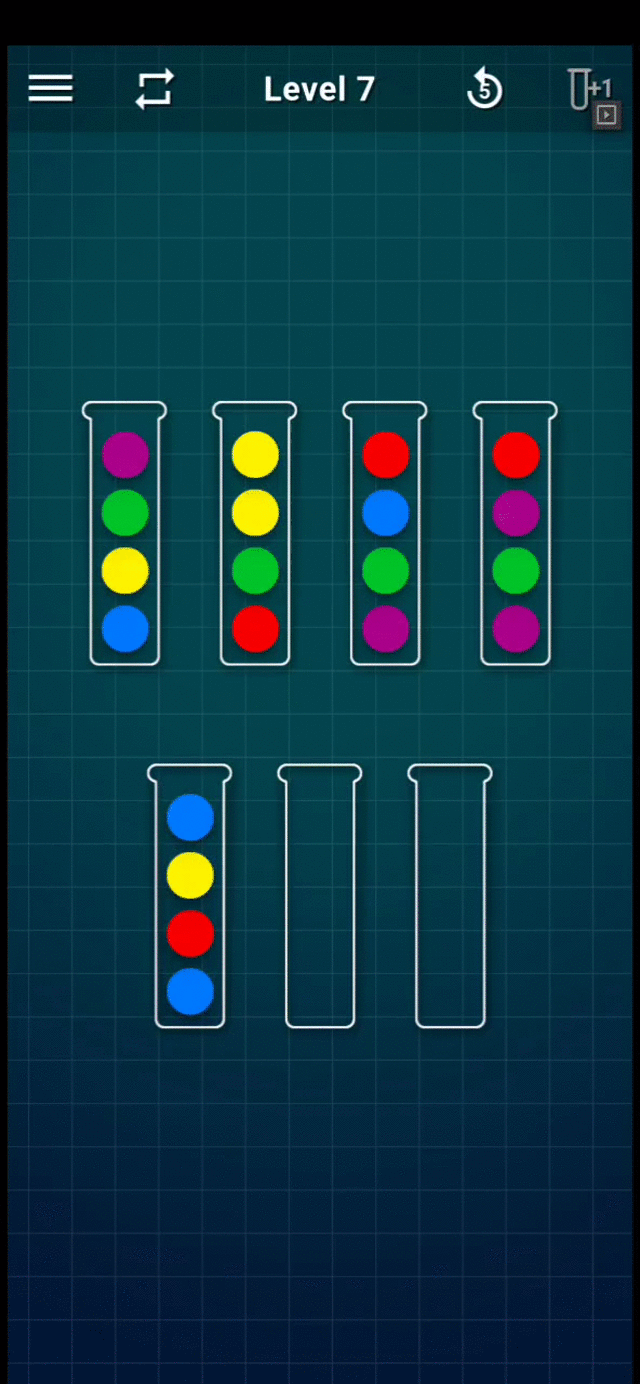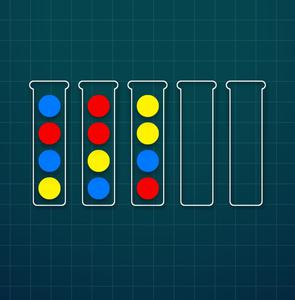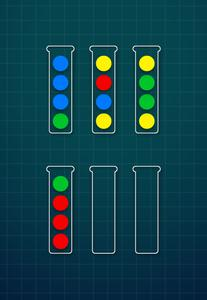Open in App
Not now

# Designing algorithm to solve Ball Sort Puzzle

• Difficulty Level : Hard
• Last Updated : 22 Nov, 2021

In Ball Sort Puzzle game, we have p balls of each colour and n different colours, for a total of p×n balls, arranged in n stacks. In addition, we have 2 empty stacks. A maximum of p balls can be in any stack at a given time. The goal of the game is to sort the balls by colour in each of the n stacks.

Rules:

• Only the top ball of each stack can be moved.
• A ball can be moved on top of another ball of the same colour
• A ball can be moved in an empty stack.

Refer to the following GIF for an example game play (Level-7):Level 7 Gameplay

Approach I [Recursion and BackTrack]:

• From the given rules, a simple recursive algorithm could be generated as below:
• Create an initial empty Queue.
• loop:
• If the current position is sorted:
• return
• else
• Enqueue all possible moves in a Queue.
• Dequeue the next move from the Queue.
• Go to loop.

However, the approach looks simple and correct, it has few caveats:

• Incorrect:
• We might end up in an infinite loop if there are >1 moves in the Queue which lead to the same position of balls.
• Inefficient:
• We might end up visiting the same position multiple times.

Thus, eliminating the above-mentioned bottlenecks would solve the issue.

Approach II [Memoization using HashMap]:

• Assumptions:
• We’ll represent ball positions as a vector of strings: {“gbbb”, “ybry”, “yggy”, “rrrg”}
• Create a set called Visited of <String> which will contain the visited positions as one long string.
• Create an empty vector for Answer which will store positions<a, b> of the tubes to move the top ball from tube a to and put it in tube b.
• Initialise grid with the initial settings of the balls.
• func solver(grid):
• loop over all the stacks (i):
• loop over all the stacks (j):
• If move i->j is valid, create newGrid with that move.
• if the balls are sorted in newGrid,
• return;
• if newGrid is NOT in Visited
• solver(newGrid)
• if solved:

Sample Game Input I:Level 3

Sample Input I:

```5
ybrb
byrr
rbyy```

Sample Output I:

```Move 1 to 4 1 times
Move 1 to 5 1 times
Move 1 to 4 1 times
Move 2 to 5 2 times
Move 1 to 2 1 times
Move 3 to 1 1 times
Move 1 to 2 1 times
Move 3 to 1 1 times
Move 2 to 1 3 times
Move 2 to 3 1 times
Move 3 to 4 1 times
Move 3 to 2 1 times
Move 2 to 4 1 times
Move 3 to 5 1 times```

Sample Game Input II:Level 5

Sample Input II:

```6
gbbb
ybry
yggy
rrrg```

Sample Output II:

```Move 1 to 5 3 times
Move 2 to 6 1 times
Move 3 to 6 1 times
Move 1 to 3 1 times
Move 2 to 1 1 times
Move 2 to 5 1 times
Move 2 to 6 1 times
Move 3 to 2 3 times
Move 3 to 6 1 times
Move 4 to 2 1 times
Move 1 to 4 1 times```

Refer to the below C++ implementation with the comments for the reference:

## C++

 `// C++ program for the above approach``#include ``using` `namespace` `std;``using` `Grid = vector;` `Grid configureGrid(string stacks[], ``int` `numberOfStacks)``{` `    ``Grid grid;``    ``for` `(``int` `i = 0; i < numberOfStacks; i++)``        ``grid.push_back(stacks[i]);` `    ``return` `grid;``}` `// Function to find the max``int` `getStackHeight(Grid grid)``{``    ``int` `max = 0;``    ``for` `(``auto` `stack : grid)``        ``if` `(max < stack.size())``            ``max = stack.size();``    ``return` `max;``}` `// Convert vector of strings to``// canonicalRepresentation of strings``string canonicalStringConversion(Grid grid)``{``    ``string finalString;``    ``sort(grid.begin(), grid.end());``    ``for` `(``auto` `stack : grid) {``        ``finalString += (stack + ``";"``);``    ``}``    ``return` `finalString;``}` `// Function to check if it is solved``// or not``bool` `isSolved(Grid grid, ``int` `stackHeight)``{` `    ``for` `(``auto` `stack : grid) {``        ``if` `(!stack.size())``            ``continue``;``        ``else` `if` `(stack.size() < stackHeight)``            ``return` `false``;``        ``else` `if` `(std::count(stack.begin(),``                            ``stack.end(),``                            ``stack)``                 ``!= stackHeight)``            ``return` `false``;``    ``}``    ``return` `true``;``}` `// Check if the move is valid``bool` `isValidMove(string sourceStack,``                 ``string destinationStack,``                 ``int` `height)``{` `    ``// Can't move from an empty stack``    ``// or to a FULL STACK``    ``if` `(sourceStack.size() == 0``        ``|| destinationStack.size() == height)``        ``return` `false``;` `    ``int` `colorFreqs``        ``= std::count(sourceStack.begin(),``                     ``sourceStack.end(),``                     ``sourceStack);` `    ``// If the source stack is same colored,``    ``// don't touch it``    ``if` `(colorFreqs == height)``        ``return` `false``;` `    ``if` `(destinationStack.size() == 0) {` `        ``// If source stack has only``        ``// same colored balls,``        ``// don't touch it``        ``if` `(colorFreqs == sourceStack.size())``            ``return` `false``;``        ``return` `true``;``    ``}``    ``return` `(``        ``sourceStack[sourceStack.size() - 1]``        ``== destinationStack[destinationStack.size() - 1]);``}` `// Function to solve the puzzle``bool` `solvePuzzle(Grid grid, ``int` `stackHeight,``                 ``unordered_set& visited,``                 ``vector >& answerMod)``{``    ``if` `(stackHeight == -1) {``        ``stackHeight = getStackHeight(grid);``    ``}``    ``visited.insert(``        ``canonicalStringConversion(grid));` `    ``for` `(``int` `i = 0; i < grid.size(); i++) {` `        ``// Iterate over all the stacks``        ``string sourceStack = grid[i];``        ``for` `(``int` `j = 0; j < grid.size(); j++) {``            ``if` `(i == j)``                ``continue``;``            ``string destinationStack = grid[j];``            ``if` `(isValidMove(sourceStack,``                            ``destinationStack,``                            ``stackHeight)) {` `                ``// Creating a new Grid``                ``// with the valid move``                ``Grid newGrid(grid);` `                ``// Adding the ball``                ``newGrid[j].push_back(newGrid[i].back());` `                ``// Adding the ball``                ``newGrid[i].pop_back();``                ``if` `(isSolved(newGrid, stackHeight)) {``                    ``answerMod.push_back(``                        ``vector<``int``>{ i, j, 1 });``                    ``return` `true``;``                ``}``                ``if` `(visited.find(``                        ``canonicalStringConversion(newGrid))``                    ``== visited.end()) {``                    ``bool` `solveForTheRest``                        ``= solvePuzzle(newGrid, stackHeight,``                                      ``visited, answerMod);``                    ``if` `(solveForTheRest) {``                        ``vector<``int``> lastMove``                            ``= answerMod[answerMod.size()``                                        ``- 1];` `                        ``// Optimisation - Concatenating``                        ``// consecutive moves of the same``                        ``// ball``                        ``if` `(lastMove == i``                            ``&& lastMove == j)``                            ``answerMod[answerMod.size() - 1]``                                     ``++;``                        ``else``                            ``answerMod.push_back(``                                ``vector<``int``>{ i, j, 1 });``                        ``return` `true``;``                    ``}``                ``}``            ``}``        ``}``    ``}``    ``return` `false``;``}` `// Checks whether the grid is valid or not``bool` `checkGrid(Grid grid)``{` `    ``int` `numberOfStacks = grid.size();``    ``int` `stackHeight = getStackHeight(grid);``    ``int` `numBallsExpected``        ``= ((numberOfStacks - 2) * stackHeight);``    ``// Cause 2 empty stacks``    ``int` `numBalls = 0;` `    ``for` `(``auto` `i : grid)``        ``numBalls += i.size();``    ``if` `(numBalls != numBallsExpected) {``        ``cout << ``"Grid has incorrect # of balls"``             ``<< endl;``        ``return` `false``;``    ``}``    ``map<``char``, ``int``> ballColorFrequency;``    ``for` `(``auto` `stack : grid)``        ``for` `(``auto` `ball : stack)``            ``if` `(ballColorFrequency.find(ball)``                ``!= ballColorFrequency.end())``                ``ballColorFrequency[ball] += 1;``            ``else``                ``ballColorFrequency[ball] = 1;``    ``for` `(``auto` `ballColor : ballColorFrequency) {``        ``if` `(ballColor.second != getStackHeight(grid)) {``            ``cout << ``"Color "` `<< ballColor.first``                 ``<< ``" is not "` `<< getStackHeight(grid)``                 ``<< endl;``            ``return` `false``;``        ``}``    ``}``    ``return` `true``;``}` `// Driver Code``int` `main(``void``)``{` `    ``// Including 2 empty stacks``    ``int` `numberOfStacks = 6;``    ``std::string stacks[]``        ``= { ``"gbbb"``, ``"ybry"``, ``"yggy"``, ``"rrrg"``, ``""``, ``""` `};` `    ``Grid grid = configureGrid(``        ``stacks, numberOfStacks);``    ``if` `(!checkGrid(grid)) {``        ``cout << ``"Invalid Grid"` `<< endl;``        ``return` `1;``    ``}``    ``if` `(isSolved(grid, getStackHeight(grid))) {``        ``cout << ``"Problem is already solved"``             ``<< endl;``        ``return` `0;``    ``}``    ``unordered_set visited;``    ``vector > answerMod;` `    ``// Solve the puzzle instance``    ``solvePuzzle(grid, getStackHeight(grid),``                ``visited,``                ``answerMod);` `    ``// Since the values of Answers are appended``    ``// When the problem was completely``    ``// solved and backwards from there``    ``reverse(answerMod.begin(), answerMod.end());` `    ``for` `(``auto` `v : answerMod) {``        ``cout << ``"Move "` `<< v + 1``             ``<< ``" to "` `<< v + 1``             ``<< ``" "` `<< v << ``" times"``             ``<< endl;``    ``}``    ``return` `0;``}`

Output

```Move 1 to 5 3 times
Move 2 to 6 1 times
Move 3 to 6 1 times
Move 1 to 3 1 times
Move 2 to 1 1 times
Move 2 to 5 1 times
Move 2 to 6 1 times
Move 3 to 2 3 times
Move 3 to 6 1 times
Move 4 to 2 1 times
Move 1 to 4 1 times```

My Personal Notes arrow_drop_up